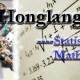## simulated annealing for SudokusOn Tuesday, Eric Chi and Kenneth Lange arXived a paper on a comparison of numerical techniques for solving sudokus. (The very Kenneth Lange who wrote this fantastic book on numerical analysis.) One of these techniques is the simulated annealing approach I had played with a long while ago.  They seem to use the same penalisation function as mine, i.e., the number of constraint violations, but the moves are different in that they pick pairs of cells without clues (i.e., not constrained) and swap their contents. The pairs are not picked at random but with probability proportional to exp(k), if k is the number of constraint violations. The temperature decreases geometrically and the simulated annealing program stops when the zero cost is achieved or when a maximum 10⁵ iterations are reached. The R program I wrote while visiting SAMSI had more options, but it was also horrendously slow! The CPU time reported by the authors is far far lower, almost in the range of the backtracking solution that serves as their reference. (Of course, it is written in Fortran 95, not in R…) As in my case, the authors mentioned they sometimes get stuck in a local minimum with only 2 cells with constraint violations.

So I reprogrammed an R code following (as much as possible) their scheme. However, I do not get a better behaviour than with my earlier code, and certainly no solution within seconds, if any. For instance, the temperature decrease in 100(.99)t seems too steep to manage 105 steps. So, either I am missing a crucial element in the code, or my R code is very poor and clever Fortran programming does the trick! Here is my code

```target=function(s){
tar=sum(apply(s,1,duplicated)+apply(s,2,duplicated))
for (r in 1:9){
bloa=(1:3)+3*(r-1)%%3
blob=(1:3)+3*trunc((r-1)/3)
tar=tar+sum(duplicated(as.vector(s[bloa,blob])))
}
return(tar)
}

cost=function(i,j,s){
#constraint violations in cell (i,j)
cos=sum(s[,j]==s[i,j])+sum(s[i,]==s[i,j])
boxa=3*trunc((i-1)/3)+1;
boxb=3*trunc((j-1)/3)+1;
cos+sum(s[boxa:(boxa+2),boxb:(boxb+2)]==s[i,j])
}

entry=function(){
s=con
pop=NULL
for (i in 1:9)
pop=c(pop,rep(i,9-sum(con==i)))
s[s==0]=sample(pop)
return(s)
}

move=function(tau,s,con){
pen=(1:81)
for (i in pen[con==0])
pen[i]=cost(((i-1)%%9)+1,trunc((i-1)/9)+1,s)
wi=sample((1:81)[con==0],2,prob=exp(pen[(1:81)[con==0]]))
prop=s
prop[wi]=s[wi]
prop[wi]=s[wi]

if (runif(1)<exp((target(s)-target(prop)))/tau)
s=prop
return(s)
}

#Example:
s=matrix(0,ncol=9,nrow=9)
s[1,c(1,6,7)]=c(8,1,2)
s[2,c(2:3)]=c(7,5)
s[3,c(5,8,9)]=c(5,6,4)
s[4,c(3,9)]=c(7,6)
s[5,c(1,4)]=c(9,7)
s[6,c(1,2,6,8,9)]=c(5,2,9,4,7)
s[7,c(1:3)]=c(2,3,1)
s[8,c(3,5,7,9)]=c(6,2,1,9)

con=s
tau=100
s=entry()
for (t in 1:10^4){
for (v in 1:100) s=move(tau,s,con)
tau=tau*.99
if (target(s)==0) break()
}
```

### 8 Responses to “simulated annealing for Sudokus ”

1.nigini Says:

Hi there.

Great work with this example.

I think I’ve might captured one (maybe the) bug in your code. When instrumented it with the same “plot” you’ve used at the first post, I could see that the “target” value was never passing some high value. Debugging the instructions inside “move” I found that value for “b” was ranging wrongly from 0..8! A plus 1 there should fix it!

Still, I’m trying to solve the “Too easy one” example but could not yet. Using t = [1:1000], tau=50, and tau=tau*0.999 I almost got it!

Regards.

•xi'an Says:

Oh dear… Thanks for spotting this mistake! Indeed, a +1 was missing in the second argument (I removed the lines with a and b as they were only used once). I am even surprised at reaching a penalty of +2 with such a mistake…

2.Useful for referring–3-25-2012 « Honglang Wang's Blog Says:

[…] simulated annealing for Sudokus […]

3.AL Says:

Perhaps this
if (runif(1)<exp((target(s)-target(prop)))/tau)
is giving numerical errors.

and
if ( tau*log(runif(1)) < (target(s) -target(prop)) )
would work better?

•xi'an Says:

Thanks. However, I do not think this is the reason, as target() never gets larger than 100 so there is no risk of overflow there…

4.AMSI lecturer « Xi'an's Og Says:

[…] (Simulation seems like a safe topic, though, as it is hard to fail to find manageable examples. Simulated annealing for sudokus was the first to come to my mind.) […]

5.AL Says:

The speed of R in loops is 100-fold (or more) lower than Fortran (specially with good compilers); and your code has loops (move) within loops (main). This is one of the main reasons people use Fortran.

•xi'an Says:

This is well-known, indeed, but it does not explain why I cannot find the solution using this code!

This site uses Akismet to reduce spam. Learn how your comment data is processed.